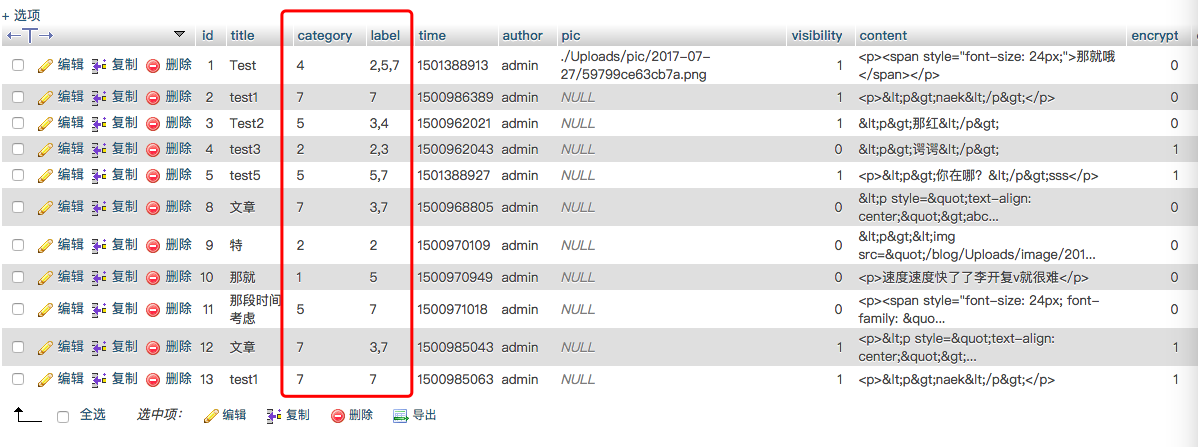﻿ TP3.2博客开发遇到的坑 - Icharle | Don't forget your first thoughts

## 视图模型&&foreach循环解决文章列表页

• 第一种方法---TP3.2中的视图模型，这个可以解决文章列表页面中的分类评论、以及评论列表页面文章标题，下面来详细说说解决的方法(就拿文章列表页面来说吧)；

当初我新建一篇文章时是直接将分类、标签的ID值存入数据库中的，为什么要怎么做？据说这样比 较高大上。视图模型的方法解决
首先我们需要在Model层中创建ArticleViewModel.class.php文件，然后在对应的控制器中(ArticleController.class.php)实例化ArticleViewModel对象，而不是Article对象。

<?php
use Think\Model\ViewModel;
class ArticleViewModel extends ViewModel {
public $viewFields = array( 'Article' =>array('*','_type'=>'LEFT'), 'Category'=>array('name'=>'cname', '_on'=>'Article.category=Category.id'), ); }  通过_on来给视图模型定义关联查询条件, Article.category为文章数据库中存该篇文章的的标签ID，而Category.id即为标签数据库中的标签ID值。cname就是为分配给模板的变量值。 • 第二种方法---利用传统的foreach循环，虽然这种方法比较笨，但是它灵活性高，适用于各种比较复杂的项目中。 这里就拿标签来做例子吧~~~~ 首先，我将标签存入数据库中是用数组的形式来存储的，因此第一步我们必须先要用foreach遍历，然后用php自带explode函数将,除去，再对其循环查找Label数据库中对应的值，再一步是又将每一篇文章的标签转成带,形式的值，最后不用说就是将其转成数轴，方便模板中循环输出。 $list = $article->order('id')->limit($Page->firstRow.','.$Page->listRows)->select();$label = D('label');
foreach($list as$value){
$l = explode(',' ,$value['label']);
$a = array();$b = array();
foreach ($l as$key => $v){$a[] = $label->id($v);

## 利用volist同时循环两个数轴

<volist name="list" id="b" key = "k">
<tr>
<td><input type="checkbox" class="input-control" name="checkbox[]" value="" /></td>
<td class="article-title">{$b.title}</td> <td>{$b.cname}</td>
<td class="hidden-sm">{$c[$k-1]}</td>
<td class="hidden-sm">{$b.commentnums}</td> <td><?php echo date('Y-m-d H:i:s',$b['time']); ?></td>
<td>
<a href="__CONTROLLER__/updatearticle/id/{$b['id']}">修改</a> <a href="__CONTROLLER__/delarticle/id/{$b['id']}" onclick="return confirm('确认删除')" >删除</a>
</td>
</tr>
</volist>


list,c为控制器中分配过来的，我们应该重点看

<td class="hidden-sm">{$c[$k-1]}</td>